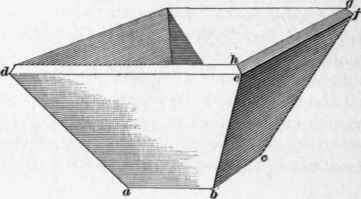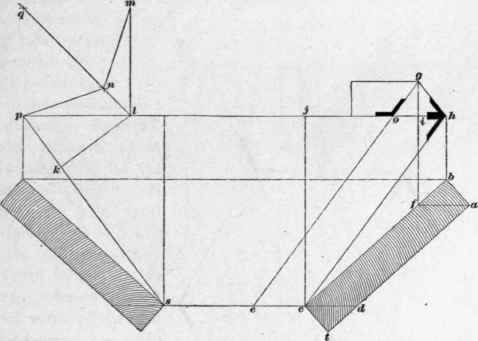Fig. 37.

72. To find the bevel for a butt joint on the square edge from a, Fig. 38, draw a f parallel to h j; from f, draw f g at right angles to h j; make i g equal to the thickness da; connect kg; then the angle j h g gives the bevel required.

To find the bevel for a miter joint on the square edge - as g h c in Fig. 37 - make c e equal to d c; parallel to c h draw e o; connect o g, then the angle j o g is the miter bevel sought; o g apparently coincides with e o prolonged, but it does not actually do so.

To find the angle between the two inside faces in a direction at right angles to the face joint p s, draw k l at any point along the line p s, and at right angles to it; at right angles to p l, draw a line through /; make l m equal to l p; with m and p as centers, describe the intersecting arcs at q; connect q l; with l k as radius, and on m and p as centers, describe intersecting arcs at n; connect n to m and to p; then m n p is the angle sought. Also, the angle p n l is the angle of miter through the thickness holding the stock of the bevel in the direction k l. A block beveled to the angle m n p will fit the angle formed by the connecting faces of the box. If either edge of the box is beveled to c d or f a, then a square line on either of these edges from the face cb will be a butt-joint line through the thickness. An angle of 45° applied from the face on either beveled edge c d or fa will also be a correct miter through the thickness.Fig. 38.

In dovetailing a box like this, instead of squaring across the ends for mortises, use the angle c f a or d a f, and, instead of squaring from the ends for the pins, use the angle c h j.

73. To find the angles required for the construction of a hexagonal box such as shown in Fig. 39: Let the hexagon (a) be the plan of the box; then draw y w at right angles to s c, one of the sides of the hexagon, and let y j be the distance over which the splay extends horizontally. Through j, parallel to s c, draw u o, and lay off j v equal to the height of the box; draw v y, and draw v z at right angles to it, and equal to the thickness of the sides; parallel to y v draw z k; draw y k perpendicular to v y; then y v z k is a cross-section of the stuff from which the box is to be made.

To find the face-joint angle, make y w equal to y v; through w, parallel to s c, draw t n; prolong the miter line a c towards r indefinitely; through o the intersection of a c prolonged and u o, draw a line parallel to y w, intersecting t w n in n, and connect n c; the face joint is then indicated by the bevel at c.

To find the angle of the butt joint to apply on the square edge v z: From s draw z x parallel to y w, and equal to z v; from x, parallel to s c, draw x m; prolong n o to m, and parallel to s c, draw z r, and connect r m; the angle required is as indicated by the bevel r. If either edge is beveled on the line y w or parallel to it, the angle of the butt joint to apply to that edge must be taken at b, while for the miter joint the angle at s must be taken.Fig. 39.

74. To find the miter for the joint of an inclined sill over an obtuse angle: Let l z t, in Fig. 40, be the angle over which the sill is to incline, and let j x and x v be lines parallel to, and equidistant from, I z and z t; then will z x be the plan of the miter line of the sill. Now draw l e at right angles to I z, and let w j be the height, j l the pitch, and l p the thickness of the sill. To find the miter angle down the face of the sill, make l e equal to l j, and parallel to z l, draw e y indefinitely; then parallel to l e draw x y, cutting e y in y, and connect y and z. The bevel at y then indicates the miter angle across the face of the sill.Fig. 40.

To find the miter angle on the square edge lp, prolong the plan miter x z towards o indefinitely, and parallel to I z draw p o. Draw o c at right angles to I z, and prolong it indefinitely; make r s equal to l p, draw s c parallel to I z, and connect c z; the bevel at c will be the angle sought If the bottom of the sill is horizontal, then I z x will be the angle of miter on that face.

75. To find the bevel for mitering the angles of a square or an octagonal pyramid: Let a b d f, Fig. 41, be the base of a square pyramid, and h j k c l m n g, the base of an octagonal pyramid. Make s e the height of the pyramid, and draw f e, de, n e, and m e; d e f will then be a side of the rectangular pyramid, and m e n a side of the octagonal one. Draw f b as the plan miter of the square base, and g c as the plan miter of the octagonal base; then, with d as a center, describe the tangent arc j i. Connect i a, and the bevel at v will indicate the angle sought for the miter of the square pyramid, while the bevel at t will indicate the miter for the sides of the octagonal pyramid. In mitering the sides of either pyramid, the bevel must be held with its stock at right angles to the edge, as shown at n.Fig. 41.

76. Window or door openings in straight walls with splayed jambs and circular splayed soffits present problems requiring an accurate knowledge of the treatment of splayed work, to secure the proper bevels and joints. To unfold the length and shape of such a window soffit on the plan of the window shown in Fig. 42, let a a', b' b be the opening, with splayed jambs a b and a' b' and circular soffit equally splayed. Continue the splay lines of each jamb a b and a' b' until they intersect at d; draw the elevation of each side of the opening as at a c a' and b f b'; divide the semicircle a c a' into any number of equal parts, say six; with d as a center, describe the arcs ag, ee' ii', and b h, all of indefinite length; on the are a g, lay off the six parts taken from a c a' and connect g to d; then ab to e' h is the length and shape of the soffit to be bent over a center after being treated by some one of the several methods hereafter explained. After the soffit is bent, the edges must be beveled as shown. Whether bent by means of keyed grooves - saw kerfed or built up and backed with staves - the grooves or staves must radiate from the center d.